# 逻辑回归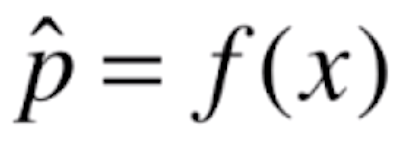，进来一个x，经过f(x)的运算，会得到一个概率值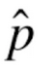。之后我们根据这个概率值来进行分类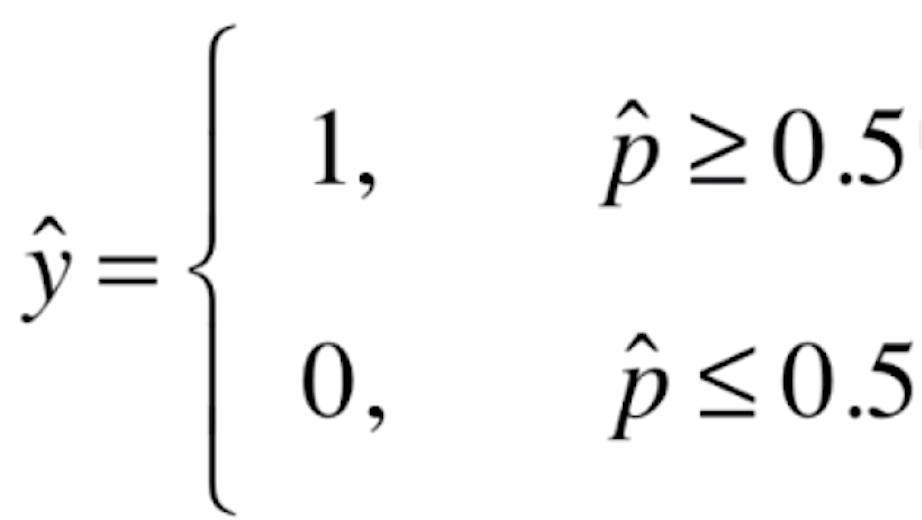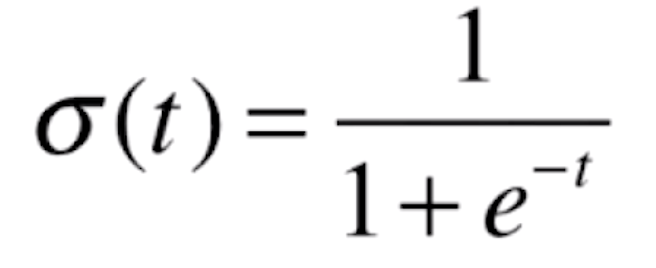```import numpy as np
import matplotlib.pyplot as plt

if __name__ == "__main__":

def sigmoid(t):
return 1 / (1 + np.exp(-t))

x = np.linspace(-10, 10, 500)
y = sigmoid(x)
plt.plot(x, y)
plt.show()```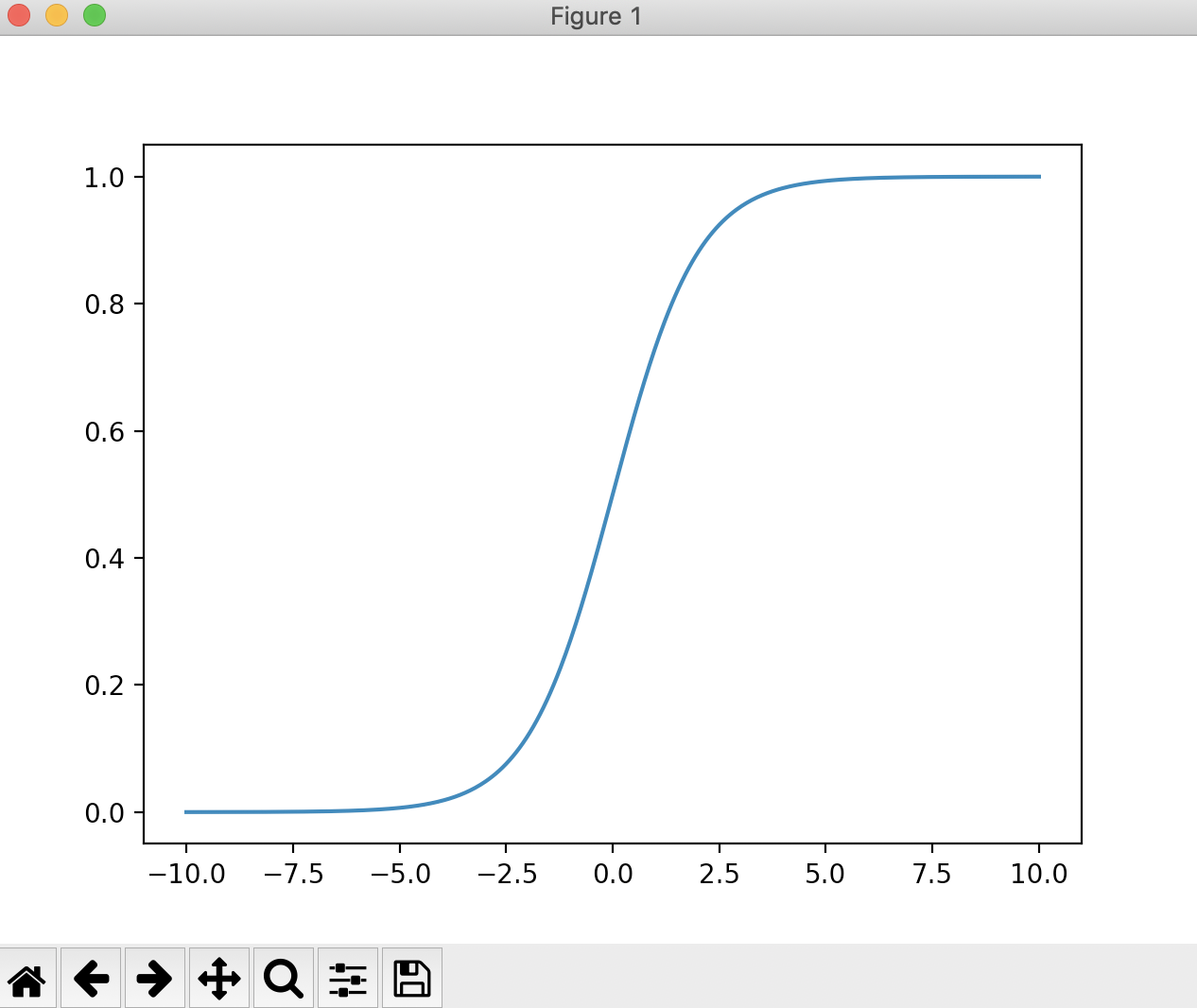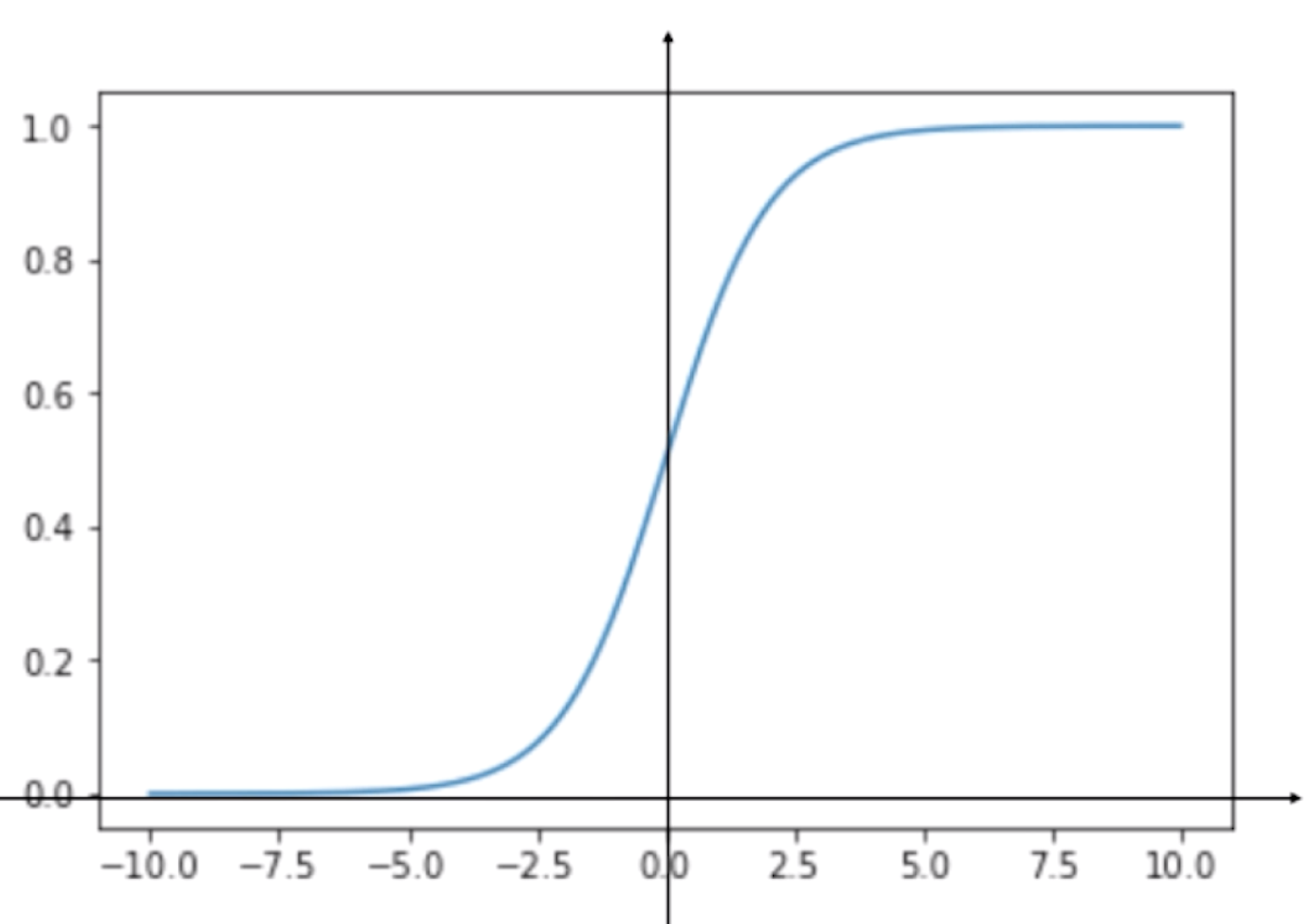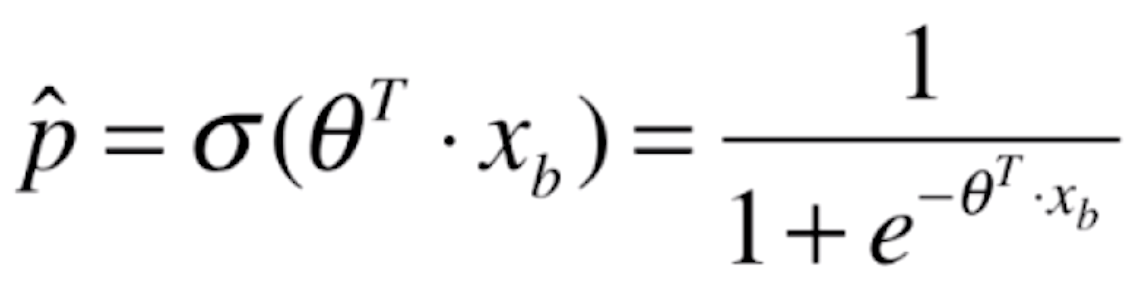「其他文章」